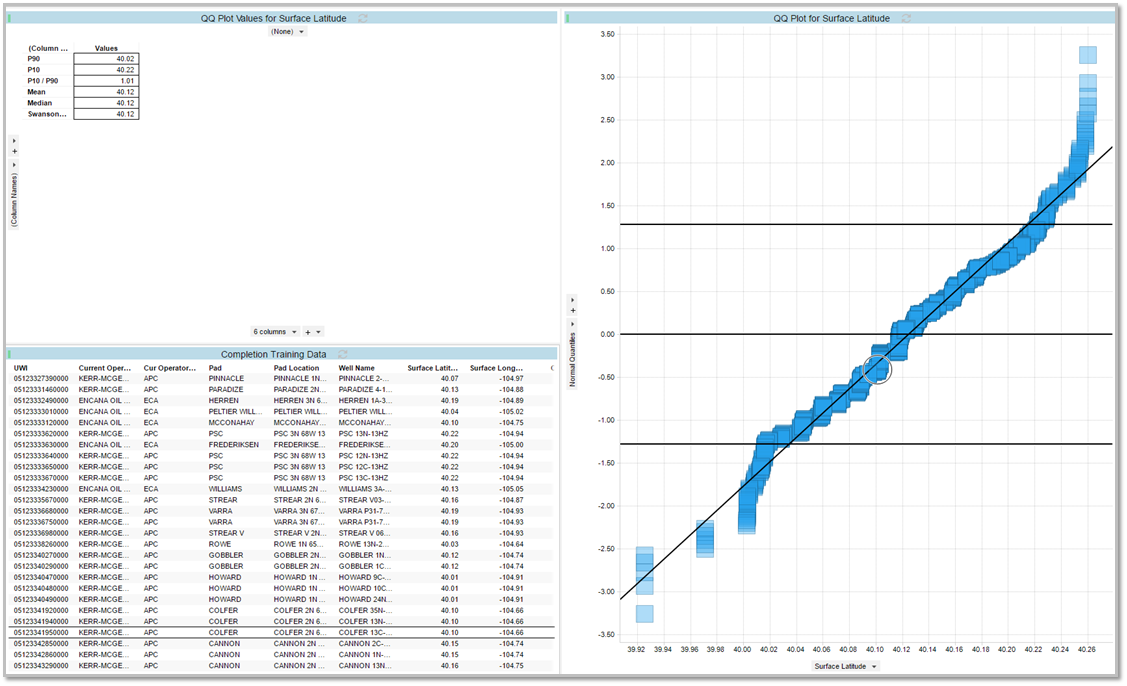## What is QQ Plot in the Data Science Toolkit?

### Purpose:

The purpose of the QQ plot is to test for normal distribution in a column of data.  Many predictive models require that the data be normally distributed.  If it is not, then the model will not work well.  This plot can help a user determine if their data is normally distributed and whether or not it should be fed into a given predictive model.

#### Output:

• Single scatter plot visualization
• Cross table for p90,p10,p10/p90, mean, median, Swanson's mean.
• Normal Quantiles are on the x-axis and the sample Quantiles are on the y-axis

Example:Data Science Toolkit QQ Plot User Guide: How to set up QQ plot

See RAI QQ Plot video below